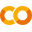# Transfer Learning with skorch¶

In this tutorial, you will learn how to train a neural network using transfer learning with the skorch API. Transfer learning uses a pretrained model to initialize a network. This tutorial converts the pure PyTorch approach described in PyTorch's Transfer Learning Tutorial to skorch.

We will be using torchvision for this tutorial. Instructions on how to install torchvision for your platform can be found at https://pytorch.org.Run in Google ColabView source on GitHub

Note: If you are running this in a colab notebook, we recommend you enable a free GPU by going:

Runtime   →   Change runtime type   →   Hardware Accelerator: GPU

If you are running in colab, you should install the dependencies and download the dataset by running the following cell:

In :
! [ ! -z "$COLAB_GPU" ] && pip install torch torchvision pillow==4.1.1 skorch ! [ ! -z "$COLAB_GPU" ] && mkdir -p datasets
! [ ! -z "$COLAB_GPU" ] && wget -nc --no-check-certificate https://download.pytorch.org/tutorial/hymenoptera_data.zip -P datasets ! [ ! -z "$COLAB_GPU" ] && unzip -u datasets/hymenoptera_data.zip -d datasets

In :
import os
from urllib import request
from zipfile import ZipFile

import torch
import torch.nn as nn
import torch.optim as optim
import numpy as np
from torchvision import datasets, models, transforms

from skorch import NeuralNetClassifier
from skorch.helper import predefined_split

torch.manual_seed(360);


## Preparations¶

In :
def download_and_extract_data(dataset_dir='datasets'):
data_zip = os.path.join(dataset_dir, 'hymenoptera_data.zip')
data_path = os.path.join(dataset_dir, 'hymenoptera_data')

if not os.path.exists(data_path):
if not os.path.exists(data_zip):
with open(data_zip, 'wb') as f:
f.write(data)

print("Starting to extract data...")
with ZipFile(data_zip, 'r') as zip_f:
zip_f.extractall(dataset_dir)


Data has been downloaded and extracted to datasets.


## The Problem¶

We are going to train a neural network to classify ants and bees. The dataset consist of 120 training images and 75 validiation images for each class. First we create the training and validiation datasets:

In :
data_dir = 'datasets/hymenoptera_data'
train_transforms = transforms.Compose([
transforms.RandomResizedCrop(224),
transforms.RandomHorizontalFlip(),
transforms.ToTensor(),
transforms.Normalize([0.485, 0.456, 0.406],
[0.229, 0.224, 0.225])
])
val_transforms = transforms.Compose([
transforms.Resize(256),
transforms.CenterCrop(224),
transforms.ToTensor(),
transforms.Normalize([0.485, 0.456, 0.406],
[0.229, 0.224, 0.225])
])

train_ds = datasets.ImageFolder(
os.path.join(data_dir, 'train'), train_transforms)
val_ds = datasets.ImageFolder(
os.path.join(data_dir, 'val'), val_transforms)


The train dataset includes data augmentation techniques such as cropping to size 224 and horizontal flips.The train and validiation datasets are normalized with mean: [0.485, 0.456, 0.406], and standard deviation: [0.229, 0.224, 0.225]. These values are the means and standard deviations of the ImageNet images. We used these values because the pretrained model was trained on ImageNet.

We use a pretrained ResNet18 neural network model with its final layer replaced with a fully connected layer:

In :
class PretrainedModel(nn.Module):
def __init__(self, output_features):
super().__init__()
model = models.resnet18(pretrained=True)
num_ftrs = model.fc.in_features
model.fc = nn.Linear(num_ftrs, output_features)
self.model = model

def forward(self, x):
return self.model(x)


Since we are training a binary classifier, the output of the final fully connected layer has size 2.

## Using skorch's API¶

In this section, we will create a skorch.NeuralNetClassifier to solve our classification problem.

### Callbacks¶

First, we create a LRScheduler callback which is a learning rate scheduler that uses torch.optim.lr_scheduler.StepLR to scale learning rates by gamma=0.1 every 7 steps:

In :
from skorch.callbacks import LRScheduler

lrscheduler = LRScheduler(
policy='StepLR', step_size=7, gamma=0.1)


Next, we create a Checkpoint callback which saves the best model by by monitoring the validation accuracy.

In :
from skorch.callbacks import Checkpoint

checkpoint = Checkpoint(
f_params='best_model.pt', monitor='valid_acc_best')


Lastly, we create a Freezer to freeze all weights besides the final layer named model.fc:

In :
from skorch.callbacks import Freezer

freezer = Freezer(lambda x: not x.startswith('model.fc'))


### skorch.NeuralNetClassifier¶

With all the preparations out of the way, we can now define our NeuralNetClassifier:

In :
net = NeuralNetClassifier(
PretrainedModel,
criterion=nn.CrossEntropyLoss,
lr=0.001,
batch_size=4,
max_epochs=25,
module__output_features=2,
optimizer=optim.SGD,
optimizer__momentum=0.9,
iterator_train__shuffle=True,
iterator_train__num_workers=4,
iterator_valid__shuffle=True,
iterator_valid__num_workers=4,
train_split=predefined_split(val_ds),
callbacks=[lrscheduler, checkpoint, freezer],
device='cuda' # comment to train on cpu
)


That is quite a few parameters! Lets walk through each one:

1. model_ft: Our ResNet18 neural network
2. criterion=nn.CrossEntropyLoss: loss function
3. lr: Initial learning rate
4. batch_size: Size of a batch
5. max_epochs: Number of epochs to train
6. module__output_features: Used by __init__ in our PretrainedModel class to set the number of classes.
7. optimizer: Our optimizer
8. optimizer__momentum: The initial momentum
9. iterator_{train,valid}__{shuffle,num_workers}: Parameters that are passed to the dataloader.
10. train_split: A wrapper around val_ds to use our validation dataset.
11. callbacks: Our callbacks
12. device: Set to cuda to train on gpu.

Now we are ready to train our neural network:

In :
net.fit(train_ds, y=None);

  epoch    train_loss    valid_acc    valid_loss    cp     dur
-------  ------------  -----------  ------------  ----  ------
1        0.8220       0.9150        0.2294     +  1.7953
2        0.4949       0.9346        0.2116     +  0.9276
3        0.4873       0.8105        0.4593        0.9309
4        0.5291       0.9477        0.1725     +  0.9292
5        0.4530       0.9216        0.2275        0.9046
6        0.3869       0.9412        0.1697        0.9121
7        0.2903       0.9608        0.1778     +  0.9504
8        0.3000       0.9477        0.1769        0.9169
9        0.4068       0.9542        0.1830        0.9312
10        0.5076       0.9281        0.1953        1.0024
11        0.3271       0.9346        0.1911        0.9144
12        0.3728       0.9281        0.2180        0.8806
13        0.2847       0.9477        0.1847        0.9466
14        0.3526       0.9216        0.2333        0.9141
15        0.3254       0.9281        0.1802        0.8951
16        0.3407       0.9477        0.1888        0.8973
17        0.2498       0.9346        0.1931        0.9159
18        0.4421       0.9477        0.1848        0.9186
19        0.3548       0.9216        0.2010        0.8960
20        0.3037       0.9281        0.2188        0.9178
21        0.3454       0.9542        0.1837        0.9184
22        0.3227       0.9412        0.1732        0.9115
23        0.2595       0.9542        0.1765        0.9040
24        0.3164       0.9477        0.1794        0.9101
25        0.2607       0.9412        0.1934        0.9493


The best model is stored at best_model.pt, with a validiation accuracy of roughly 0.96.

Congrualations! You now know how to finetune a neural network using skorch. Feel free to explore the other tutorials to learn more about using skorch.